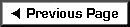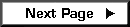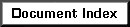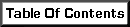A shear wall is only as strong as the load path leading to it. Loads must be collected in other portions of the structure and delivered to the top of the shear wall so they can be transferred into the plywood sheathing. Often this is accomplished by means of the wall's double top plate.

Double 2x plates are stacked, lapped and nailed together. The number and size of nails determines the strength of the connection. In rare instances - mostly where 2 x 4 plates are used - the strength of the wood may have a bearing.

An example of a collector is presented below for your reference. The steps used to calculate the maximum collector force are applicable to similar elements constructed from beam segments or combinations of wood and steel plates. The assumption is that the element that delivers load to the collector is continuously connected to it ( a roof or second floor ).

 Example of a Collector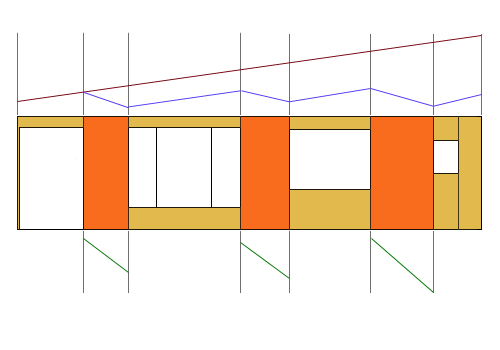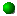The wall sections that resist shear are shaded orange.  All other sheathing is tan. The total building length is 45 feet. The walls are, from left to right, 4 feet, 5 feet and 6 feet long. The window regions, from left to right are 6 feet, 12 feet, 8 feet and two feet with a 2 foot long segment of wall at the right. The total shear force in this wall line is 4500 pounds.

The total lateral force "F" is apportioned to walls A (at left), B (in center) and C (at right) according to their length.  The 2 foot long wall at the far right does not meet the minimum width criteria set by the current Code. Total useful wall length = 15 feet.

Force to wall A = (4500)(4/15) = 1,200 lbs.

Force to wall B = (4500)(5/15) = 1,500 lbs.

Force to wall C = (4500)(6/15) = 1,800 lbs.

Note these are all equal to 300 pounds per foot.  Think of the walls as removing 300 pounds per foot from the system. The negative slope of the green lines above indicate loads are being removed load from the wall system.

The force delivered to the collector is (4500)/(45) = 100 pounds per foot.

Note that the red line above has a positive slope.  It indicates load is being contributed to the system.

The blue line represents the net collector force at any point along the wall length. It slopes down at 200 pounds per foot in the wall regions and climbs at 100 pounds per foot in the window regions. The collector force can be read from a scale graph of these slopes or calculated as follows:

• Starting at the left, the force in the collector is zero. But force builds up over 6 feet until we enter the first wall region. The collector force at this location is (6)(100) = 600 pounds.
• Over the next 4 feet we will continue to add 100 pounds per foot for a total of 1000 pounds but we will also be deducting 300 pounds per foot (-1200 lbs.). The net result at the right edge of the 4 foot shear wall is a collector force of -200 pounds.
• To continue, we will add 100 pounds per foot for 12 feet for a net collector force of +1000 pounds.
• In the next 5 feet we will add 500 pounds but also reduce by 1500 pounds leaving a net collector force at the right edge of zero pounds.
• The remainder are: +800, -400.

We would design this collector line to resist a maximum force of 1000 pounds. Using 16d common nails…

(1000 lbs.) / (141 * 1.33 * (1.5/1.78)) = 6.33 nails >> Use 7-16d's minimum staggered and spaced 4" o.c.

If you would like to try your hand at collectors, use the same forces as above but eliminate wall B.